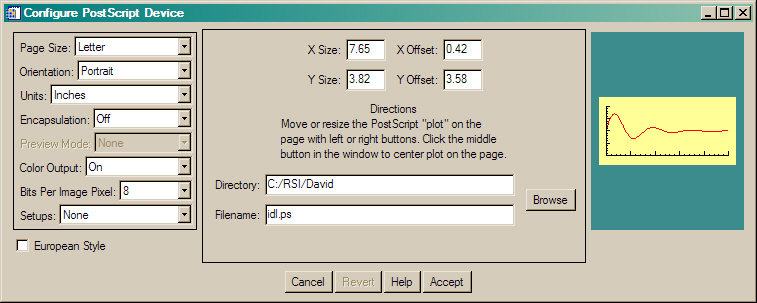PostScript Output that Looks Like Display Output

QUESTION: My PostScript output looks elongated (squished, strange, etc.). How can I make it look like the output on my display?ANSWER: The PostScript output window rarely--if ever--has the same aspect ratio as your display window. If you don't know this, you will have a hard time getting your PostScript output to look like your display output.

The PostScript output window is set up with the XSIZE and YSIZE keywords to the DEVICE command. By default this window is 7 inches in the X direction and 5 inches in the Y direction in Portrait mode. This is rarely the aspect ratio (ratio of window height to width) of your display window. If you issue a graphics command like PLOT or SURFACE or CONTOUR, IDL normally "fills" the output window with the graphical output. Thus, the plot you see on your display will have a different aspect ratio then the plot you see on the PostScript page.

You have several choices for how to deal with this problem.

In the simplest case, you can make your display windows and your PostScript windows have the same aspect ratio. For example, you can make them square, like this:

Window, XSIZE=500, YSIZE=500
PLOT, data

or, in PostScript:

SET_PLOT, 'PS'
DEVICE, XSIZE=5, YSIZE=5, /INCHES
PLOT, data

A more general solution is to configure your PostScript output window with the same aspect ratio as the current display window. For example, suppose you want to print a PostScript page in portrait mode and you want the plot to have the same aspect ratio is has on your display. Here is an example of how you might calculate the correct size for your PostScript output window. Note that I have reduced the full page size by one-half inch. This allows the plot to be printed on most PostScript printers.

; Calculate the aspect ratio of display window.
aspectRatio = FLOAT(!D.Y_VSIZE) / !D.X_VSIZE

; Now calculate the correct size on a Portrait page.
xsize = 8.0
ysize = xsize * aspectRatio
IF ysize GT 10.5 THEN BEGIN
ysize = 10.5
xsize = ysize / aspectRatio
ENDIF

; Calculate the offsets, so the output window is not off the page.
xoffset = (8.5 - xsize) / 2.0
yoffset = (11.0 - ysize) / 2.0

; Configure the PostScript device.
SET_PLOT, 'PS'
DEVICE, XSIZE=xsize, YSIZE=ysize, XOffset=xoffset, YOffset=yoffset

Fortunately, it is quite easy to make this kind of code general. In fact, it has already been done for you. I use the program PSWINDOW to calculate the size of a PostScript window that has the same aspect ratio of the current display window. The program returns a structure with XSize, YSize, XOffset, and YOffset fields set to the largest PostScript window that maintains the aspect ratio of the display window. It works like this:

a = PSWINDOW()
SET_PLOT, 'PS'
DEVICE, XSIZE=a.xsize, YSIZE=a.ysize, XOFFSET=a.xoffset, \$
YOFFSET=a.yoffset, /INCHES

Or, more likely, you just you keyword inheritance to configure the PostScript device.

keywords = PSWINDOW()
SET_PLOT, 'PS'
DEVICE, _EXTRA=keywords

Normally, PSWINDOW returns sizes and offsets in inches and uses an American 8.5x11.0 inch page size. But, you can set the METRIC keyword, and the sizes can then be expressed in centimeters and an A4 page size will be used.

keywords  = PSWINDOW(/LANDSCAPE, /METRIC)
SET_PLOT, 'PS'
DEVICE, _EXTRA=keywords

I usually just configure the PostScript device all at once with PSConfig.

Window, XSize=500, YSize=250
keywords = PSConfig(_Extra=PSWindow())
DEVICE, _EXTRA=keywords

Here is what PSConfig looks like when it comes up. Notice the “window” on the PostScript page (on the right) has an aspect ratio of 2:1.PSConfig is set up with a “window” having the same aspect ratio as the current graphics window.

This is how the Coyote Graphics routines manage to automatically produce PostScript output that looks identical to what is shown on the display.Setting the Aspect Ratio

Sometimes I have the opposite problem. I want a plot to have a particular aspect ratio, but I don't know or care what size the window will be. This is the case, for example, with resizeable graphics windows. In this case, I use the program cgASPECT, which calculates and returns the position coordinates in the current graphics window of a plot with a particular aspect ratio. It works as well in PostScript as it does on the display.

For example, suppose I want a plot that is twice as wide as it is tall. It has an aspect ratio of 1:2 or 0.5. If I want to know how to place that plot in a window of unknown dimensions, I do this:

plotPosition = cgASPECT(0.5)
PLOT, data, POSITION=plotPosition